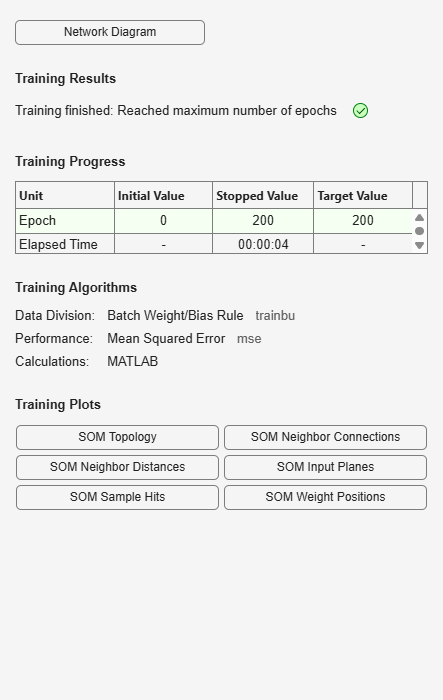# plotsomnd

Plot self-organizing map neighbor distances

## Syntax

```plotsomnd(net) ```

## Description

`plotsomnd(net)` plots a `SOM` layer showing neurons as gray-blue patches and their direct neighbor relations with red lines. The neighbor patches are colored from black to yellow to show how close each neuron’s weight vector is to its neighbors.

This plot supports `SOM` networks with `hextop` and `gridtop` topologies, but not `tritop` or `randtop`.

## Examples

### Plot SOM Neighbor Distances

```x = iris_dataset; net = selforgmap([5 5]); net = train(net,x);````plotsomnd(net)`## Version History

Introduced in R2008a